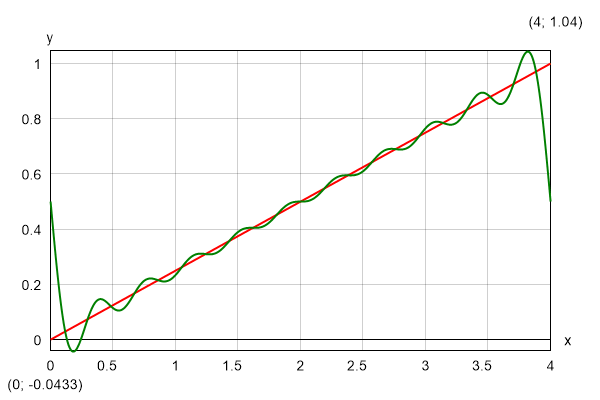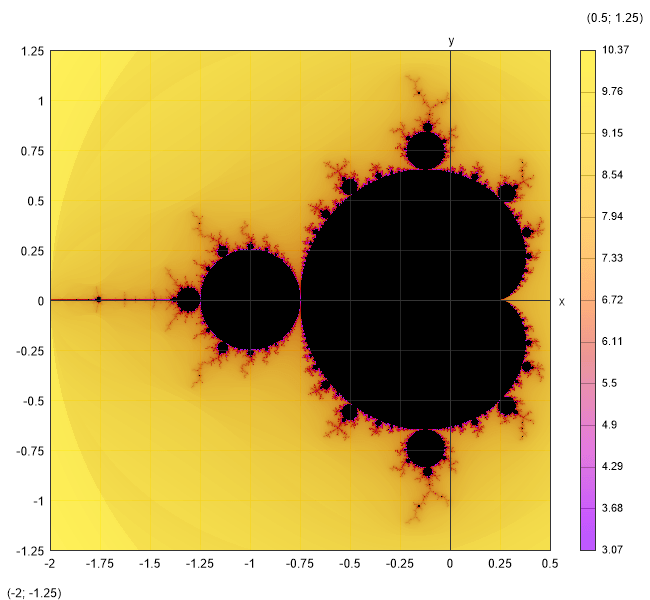Topic: Expressions

## Iterative procedures

There are some other commands that allows you to calculate the result iteratively. Unlike numerical methods, they can work with complex numbers.

#### Sum

`\$Sum{f(k) @ k = a : b}`

It sums the values of f(k) for all integer k between a and b. The values of k can only grow, so it should be satisfied that a < b. Instead of f(k) you can put any valid expression that includes k. Otherwise, it will simply sum the same value k times. For example, you can use series to calculate constants. Such is the Leibniz formula for calculation of π:

`4*\$Sum{(-1)k+1/(2*k - 1) @ k = 1:1000}= 3.1406`

You can also use series to define functions. Of course, they cannot be infinite. The number of iterations should be sufficient to provide the required precision of the result. The following pattern can be applied to approximate a function with Fourier series:

`f(x) = a0/2 + \$Sum{a(k)*cos(k*x*π/l) @ k = 1:n} + \$Sum{b(k)*sin(k*x*π/l) @ k = 1:n}`

As an example, we can take a straight line within the interval (0; 2*l), withs equation: f(x) = x/(2*l). The integration constants are a(k) = 0 and b(k) = -1/(k*π). If we plot the Fourier approximation for n = 5, we will get the following result:#### Product

`\$Product{f(k) @ k = a : b}`

It works like "Sum", but it multiplies the terms instead of adding them. For example, you can define your own factorial function:

`F(n) = \$Product {k @ k = 1 : n}`

You can use it further to calculate binomial coefficients by the well-known formula: C(n; k) = F(n)/(F(k)*F(n - k)). However, it is much more efficient to define a special procedure that computes the coefficient directly without using factorials:

`\$Product{(i + n - k)/i @ i = 1:k}`

Also, the later will not overflow together with the factorials for greater values of n.

#### Repeat

`\$Repeat{f(k) @ k = a : b}`

This is a general inline iterative procedure that repeatedly calculates f(k). It can be used for sums and products instead of the respective procedures, but it is not so efficient. However, there are expressions that can be calculated only by the "Repeat" command. Normally, such expressions will make sense if you assign the result to a variable to be used in the next iteration. So, the following pattern is more likely to be applied in practice:

`\$Repeat{x = f(x; k) @ k = a : b}`

For example, you can use this command to define the Mandelbrot set in a single line:

`f(z; c) = \$Repeat{z = z^2 + c @ i = 1:100}`

You should not forget to switch to "Complex" mode. Then you can plot the result:

`\$Map{abs(f(0; x + 1i*y)) @ x = -1.5:0.5 & y = -1:1}`